# 理解 Word Embedding，全面拥抱 ELMO## 1. 理解 Word Embedding

### 1.1 图像预训练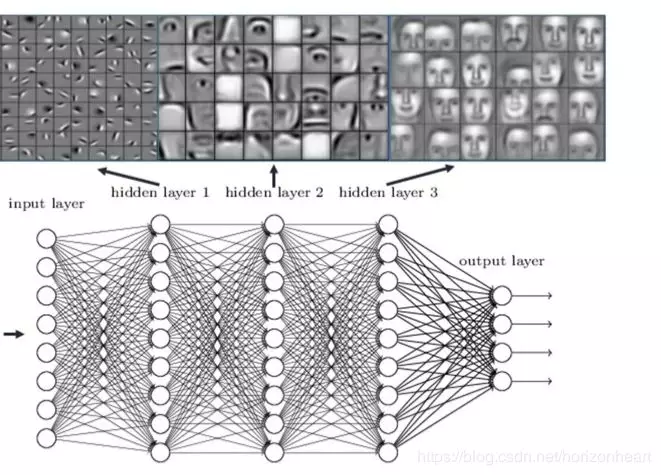### 1.2 Word Embedding 预处理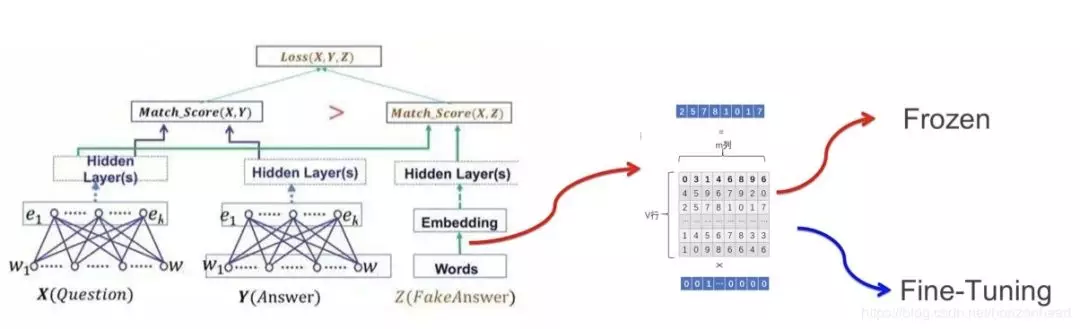## 2. 理解 ELMO

### 2.1 理论

ELMO 使用了双向循环神经网，包含了一个正向的和一个反向的 LSTM 。如图：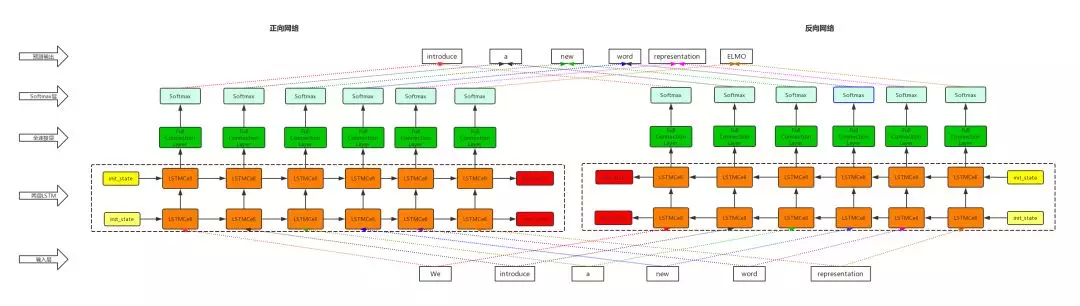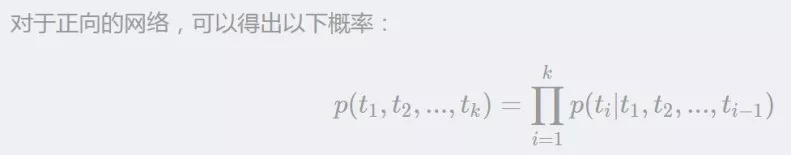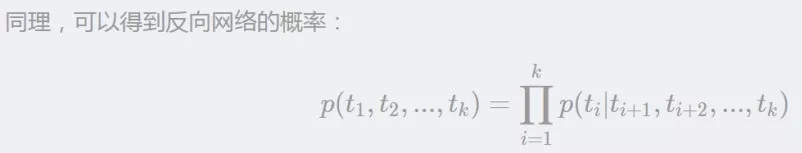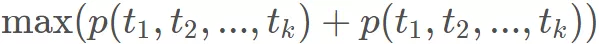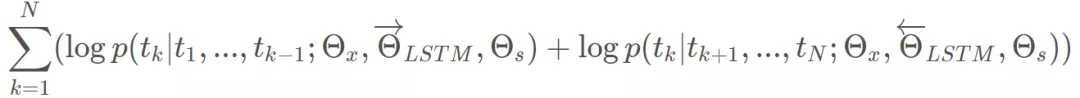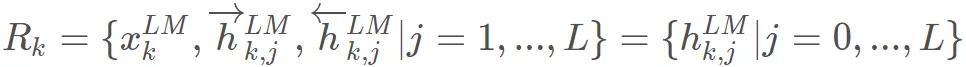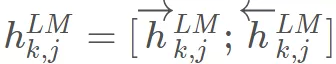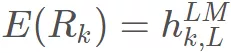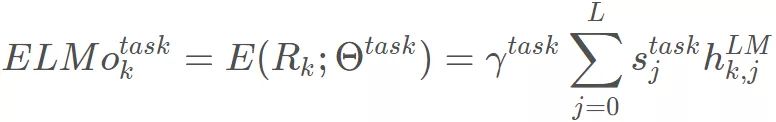${s}_{j}$$s_j$ 是一个 softmax 出来的结果，$\gamma$$\gamma$ 是任务相关的 scale 参数。

Pre-trained 的 language model 是用了两层的 LSTM ，对 token 进行上下文无关的编码是通过 CNN 对字符进行编码, 然后将三层的输出 scale 到 1024 维，最后对每个 token 输出 3 个 1024 维的向量表示。这里之所以将 3 层的输出都作为 token 的 embedding 表示是因为实验已经证实不同层的 LSTM 输出的信息对于不同的任务作用是不同的, 也就是所不同层的输出捕捉到的 token 的信息是不相同的。此时得到 token 的 embedding vector ，就包含了 context 的信息了。

### 2.2 代码分析

https://github.com/allenai/bilm-tf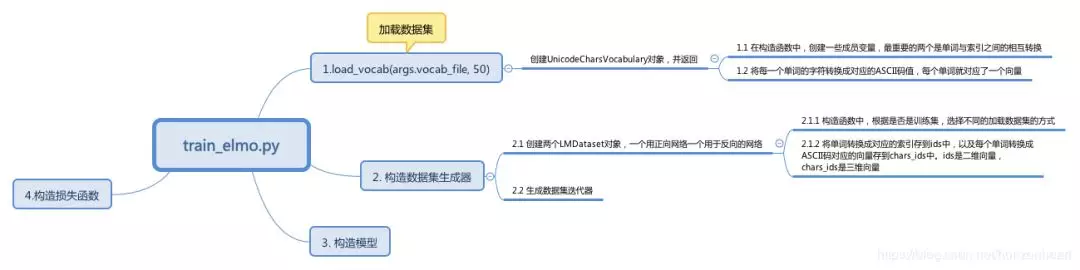#### 2.2.1 加载 vocabulary

#加载 vocabularydef load_vocab(vocab_file, max_word_length=None):if max_word_length:return UnicodeCharsVocabulary(vocab_file, max_word_length,                                      validate_file=True)else:return Vocabulary(vocab_file, validate_file=True)

#### 2.2.2 构造数据集生成器

def _get_batch(generator, batch_size, num_steps, max_word_length):    """Read batches of input."""    cur_stream = [None] * batch_size      no_more_data = False    while True:        #todo 输入大小        inputs = np.zeros([batch_size, num_steps], np.int32)        if max_word_length is not None:            #todo 每个单词的输入，将单词转化成数字            char_inputs = np.zeros([batch_size, num_steps, max_word_length],                                np.int32)        else:            char_inputs = None          targets = np.zeros([batch_size, num_steps], np.int32)          for i in range(batch_size):            cur_pos = 0              while cur_pos < num_steps:                if cur_stream[i] is None or len(cur_stream[i]) <= 1:                    try:                        cur_stream[i] = list(next(generator))                    except StopIteration:                        # No more data, exhaust current streams and quit                        no_more_data = True                        break                #todo  这个地方减一是为了构造 target                how_many = min(len(cur_stream[i]) - 1, num_steps - cur_pos)                next_pos = cur_pos + how_many                  inputs[i, cur_pos:next_pos] = cur_stream[i][:how_many]                if max_word_length is not None:                    char_inputs[i, cur_pos:next_pos] = cur_stream[i][                                                                    :how_many]                #todo 构造 target 目标                targets[i, cur_pos:next_pos] = cur_stream[i][1:how_many+1]                  cur_pos = next_pos                  cur_stream[i] = cur_stream[i][how_many:]                if max_word_length is not None:                    cur_stream[i] = cur_stream[i][how_many:]          if no_more_data:            # There is no more data.  Note: this will not return data            # for the incomplete batch            break          X = {'token_ids': inputs, 'tokens_characters': char_inputs,                 'next_token_id': targets}          yield X

num_steps 是序列的长度，也就是我们用于训练 RNN 的时候，考虑了几个时间步长。

#### 2.2.3 构造模型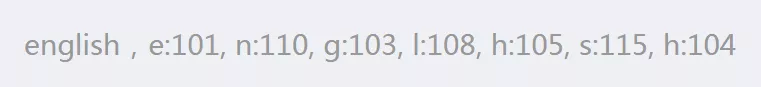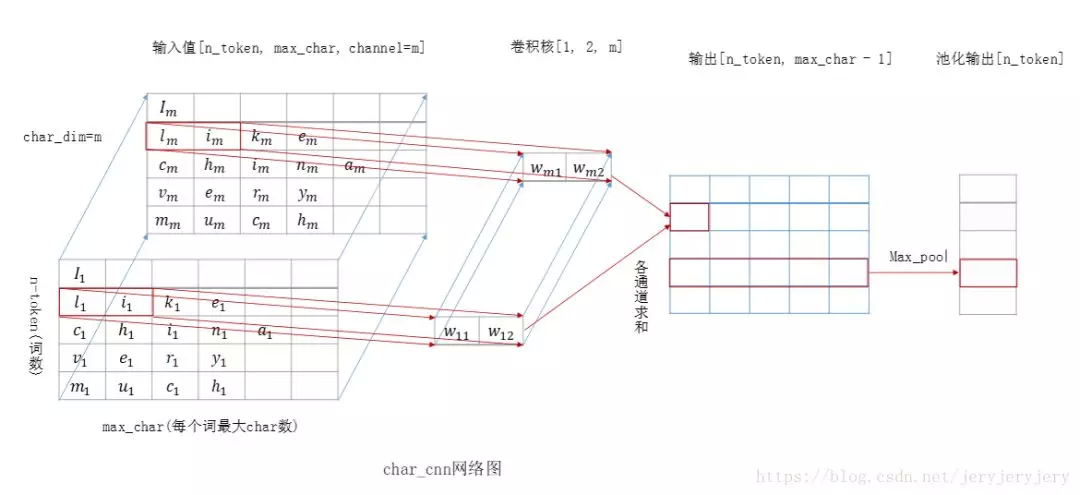#todo #字符的输入 self.tokens_characters = tf.placeholder(DTYPE_INT,                             shape=(batch_size, unroll_steps, max_chars),                             name='tokens_characters')  # the character embeddings  with tf.device("/cpu:0"):      #todo 字符的 embedding 矩阵      self.embedding_weights = tf.get_variable(              "char_embed", [n_chars, char_embed_dim],              dtype=DTYPE,              initializer=tf.random_uniform_initializer(-1.0, 1.0)      )      # shape (batch_size, unroll_steps, max_chars, embed_dim)      # todo 构造输入数据      self.char_embedding = tf.nn.embedding_lookup(self.embedding_weights,                                              self.tokens_characters)

#### 2.2.4 其它

https://github.com/horizonheart/ELMO

https://mp.weixin.qq.com/s/zfoQd-IOpTU-qCEuRHTU_A## 最新评论WEI WEI 2019 年 07 月 26 日 06:14 0 回复